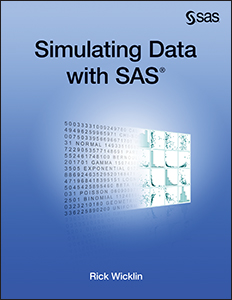Data simulation is a fundamental technique in statistical programming and research. My book Simulating Data with SAS is an accessible how-to book that describes the most useful algorithms and the best programming techniques for efficient data simulation in SAS. Here are five lessons you can learn by reading it:

• Learn strategies for designing a simulation study. What is the best what to vary parameters in a simulation study? A poorly designed study can result in many wasted hours of computation. This book provides general advice and illustrates it with specific programs.
• Learn to use elementary probability distributions to simulate from more complex distributions. SAS software provides an easy way to simulate data from about 30 standard probability distributions, but what if you need a more complicated model? The book shows how to use the standard distributions to simulate data from complex distributions.
• Learn how to simulate data that follow a regression model. The book shows you how to simulate response variables for general linear regression models, generalized linear models, mixed models, longitudinal models, time series models, and more.
• Learn efficient techniques that save time. Yes, simulation is a brute-force statistical method, but efficiency matters. The book describes tips and techniques that can decrease the computational time a hundredfold. If your simulation currently requires hours, you might be able to rewrite it to run in seconds.
• Learn to use SAS/IML software for simulations that involve multivariate data. Using the right tool makes completing a task faster and simpler. Although it is possible to turn screws with a pair of pliers, that tool is not optimal for that task. Similarly, although the DATA step is a powerful tool for some simulation tasks, the SAS/IML matrix language is a better tool for complex simulations and for those that involve multivariate data. I introduce the SAS/IML language gradually and show you how to complete many tasks by using either tool.

Simulation is a powerful statistical technique. Whether you are a novice or an expert, I hope that you will find many tips in Simulating Data with SAS that enable you to write simulations that are both effective and efficient.

ShareDistinguished Researcher in Computational Statistics

Rick Wicklin, PhD, is a distinguished researcher in computational statistics at SAS and is a principal developer of SAS/IML software. His areas of expertise include computational statistics, simulation, statistical graphics, and modern methods in statistical data analysis. Rick is author of the books Statistical Programming with SAS/IML Software and Simulating Data with SAS.

1.I have multiple data sets named
dsnAC16 dsnAC17 dsnAC18 dsnAC19 dsnAC110.........dsnAC125
dsnAC26 dsnAC27 dsnAC28 dsnAC29 dsnAC210.........dsnAC225
.
.
.
dsnAC56 dsnAC57 dsnAC58 dsnAC59 dsnAC510.........dsnAC525

I want to combine all above data set without macro loop and I am using following code but getting some errors pls guide

%let i=5; %let pat=20;

data combineAC;
set
do %let k = 1 to &i;

do %let h= %eval(1+&i) to %eval(&pats+&i);

dsnAC&k&h
end;
end;

run;

•1) I assume you are doing a simulation study. You don't need to create those individual data set. See the article Simulation in SAS, or see my book.

2) If you insist of concatenating data sets, use
SET dsnAC:;

3) If you absolutely need to use a macro (you don't), here's the correct syntax for the %DO iterative statement..